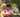### Binary Gap

January 16, 2020

Binary Gap

JavaScript Tech Interview Exercise

Write a function:
function solution(N);
that, given a positive integer N, returns the length of its longest binary gap. The function should return 0 if N doesn't contain a binary gap. For example, given N = 1041 the function should return 5, because N has binary representation 10000010001 and so its longest binary gap is of length 5. Given N = 32 the function should return 0, because N has binary representation '100000' and thus no binary gaps.

First we need to convert the integer into binary representation in '0' or '1' using Number.toString() or write a function like getBinaryBase2(n).

``````function getBinaryBase2(n){
let result = ''
while (n>0){
result = (n % 2) + result
n = Math.trunc( n/2 )
}
return result
}``````

Next step is to find the first '1', set the start flag to true, and then start counting '0'. When finding the next '1', save the zero counts to an array bgap[] and reset the count.

Final step is to find & return the maxium value in bgap[].

My solution :

``````function solution(N) {
// write your code in JavaScript (Node.js 8.9.4)
const str = N.toString(2) //convert an integer to binary representation

let bgap = []
let bindex = 0
let start = false
let count = 0

for(let d of str){
if( d === '1') {
if(!start) start = true
if(start){
bgap[bindex] = count
bindex += 1
count = 0    // reset
}
} else { // d === '0'
if(start) count += 1
}
}

if(bgap.length<1) return 0 // no binary gap

let max = 0
for(let i=0; i<bgap.length; i++){
if(bgap[i]>max) max=bgap[i]
}
return max
}

console.log("binary gap: ", solution(529))``````Posted by Tai Lu ( 呂台生 ) who's building useful things.You should follow him on Twitter